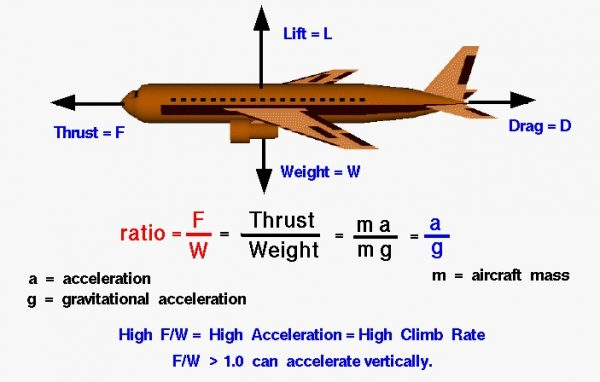# Thrust to Weight Ratio## Four Forces

There are four forces that act on an aircraft in flight: lift, weight, thrust, and drag. Forces are vector quantities having both a magnitude and a direction. The motion of the aircraft through the air depends on the relative magnitude and direction of the various forces. The weight of an airplane is determined by the size and materials used in the airplane’s construction and on the payload and fuel that the airplane carries. The weight is always directed towards the center of the Earth.

The thrust is determined by the size and type of propulsion system used on the airplane and on the throttle setting selected by the pilot. Thrust is normally directed forward along the centerline of the aircraft. Lift and drag are aerodynamic forces that depend on the shape and size of the aircraft, air conditions, and the flight velocity. Lift is directed perpendicular to the flight path and drag is directed along the flight path.

## Force Equation

Just as the lift to drag ratio is an efficiency parameter for total aircraft aerodynamics, the thrust to weight ratio is an efficiency factor for total aircraft propulsion. From Newton’s second law of motion for constant mass, force F is equal to mass m times acceleration a:

## Weight Equation

If we consider a horizontal acceleration and neglect the drag, the net external force is the thrust F.

From the Newtonian weight equation:

### W = m * g

where W is the weight and g is the gravitational constant equal to 32.2 ft/sec^s in English units and 9.8 m/sec^s in metric units. Solving for the mass:

## Mass Equation

### m = W / g

and substituting in the force equation:

## Thrust to Weight Ratio

F/W is the thrust to weight ratio, and it is directly proportional to the acceleration of the aircraft. An aircraft with a high thrust to weight ratio has high acceleration. For most flight conditions, an aircraft with a high thrust to weight ratio will also have a high value of excess thrust. High excess thrust results in a high rate of climb. If the thrust to weight ratio is greater than one and the drag is small, the aircraft can accelerate straight up like a rocket. Similarly, rockets must develop thrust greater than the weight of the rocket in order to lift off.

NOTE: We must be very careful when using data concerning the thrust to weight ratio. Because airframes and engines are produced by different manufacturers and the same engine can go into different airframes, the thrust to weight ratio of the engine alone is often described in the literature. High thrust to weight is an indication of the thrust efficiency of the engine. But when determining aircraft performance, the important factor is the thrust to weight of the aircraft, not just the engine alone. Another problem occurs because the thrust of an engine decreases with altitude while the weight remains constant. Thrust to weight ratios for engines are often quoted at sea level static conditions, which give the maximum value that the engine will produce.

Provide feedback